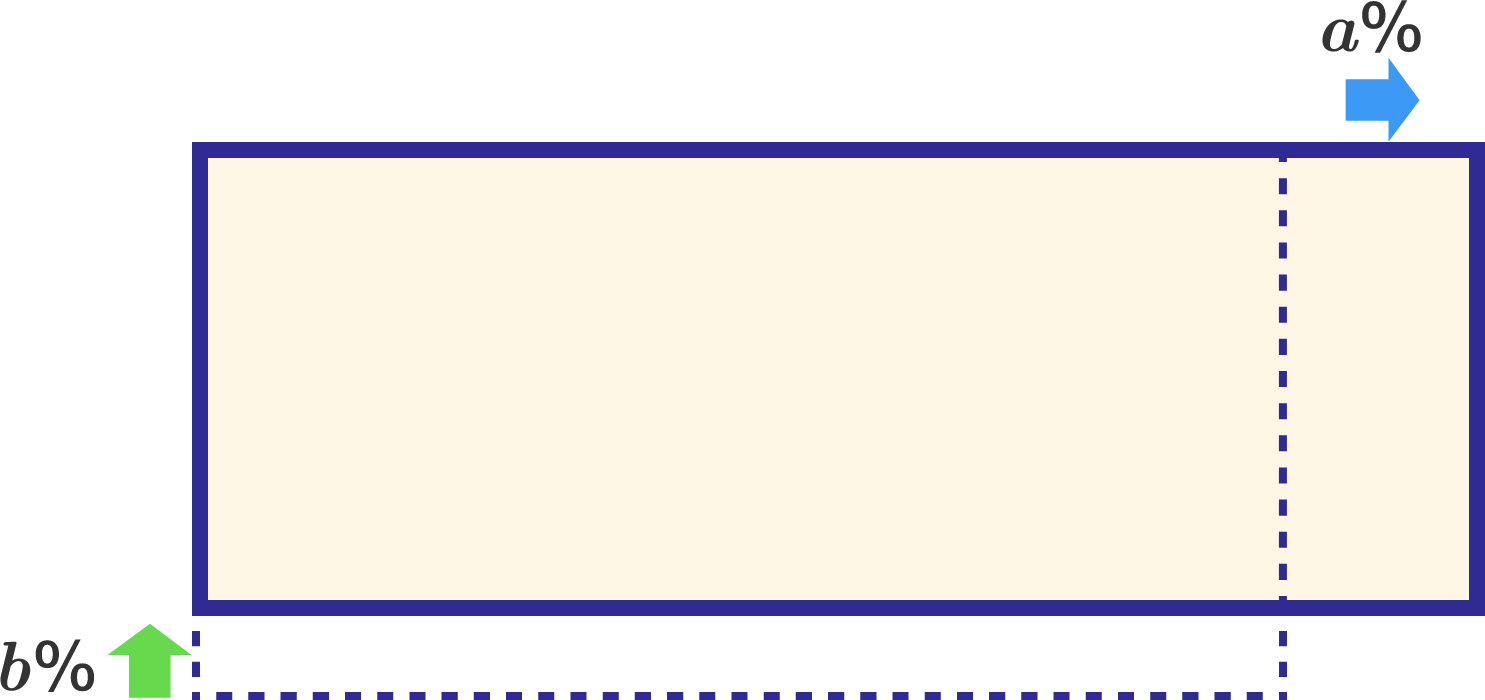Geometry Level 4

Let $a$ and $b$ be positive integers less than 100.

If the length of a certain rectangle is increased by $a\%$, and the width of the same rectangle is decreased by $b\%$, then the resultant area is increased by $20.17\%$.

Find $a+b$.×